Maths-
General
Easy

Question

# Factor the polynomial as the product of binomials.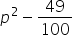Hint:

## The correct answer is: (p-7/10)(p+7/10) is the required product of binomialseses open parentheses p plus 7 over 10 close parentheses is the required product of binomials.

### Ans :-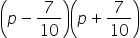is the required product of binomials.Given ,Write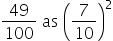then we get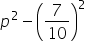As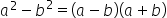Here a = p and b =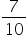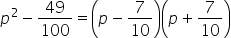∴is the required product of binomials.#### With Turito Foundation.#### Get an Expert Advice From Turito.